Sulfur-Sulfate Trends for IMPROVE

Author:  Robert Eldred, CNL, University of California, Davis (raeldred@ucdavis.edu)

Date:  May 17, 2001

Summary:  Warren White noted a trend in the ratio of nylon sulfate / Teflon sulfur over the course of the IMPROVE network.  This is extremely critical in determining sulfate trends.  This report concludes that there is no trend in the ratio of analytical calibrations, but there is a trend in the relationship at low concentrations.  This is seen in the intercept and in distributions.  The low end concentrations are primarily affected by the artifact that is subtracted from the samples.  This change in low end concentrations can account for the trend in the ratios.  No mechanism for producing such a change has been identified.

Background

Sulfur is measured on Teflon filters using Proton Induced X-ray Emission, a variant of X-Ray Fluorescence.  Because the sulfur measured on field blanks is near the analytical minimum detectable limit, no artifact is subtracted from the measured sulfur.  The sulfur concentrations are multiplied by 3 and labeled S3.  Sulfate is measured by Ion Chromatography on nylon filters.  An artifact is determined from the field blanks collected during the season.

Figure 1 shows the ratios at an Eastern site (Acadia National Park) and a Western site (Bryce Canyon National Park).  The highest ratio at Acadia is associated with a loss in sulfur from Teflon at Eastern sites in the mid 1990’s.  The high ratios at Bryce Canyon are all associated with very lightly loaded samples.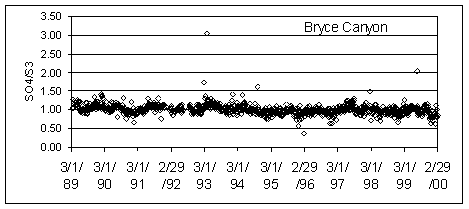Figure 1.  SO4/S3 ratios at Acadia and Bryce Canyon National Parks.

Warren White minimized the scatter by examining running annual median concentrations.  In this approach, the annual median centered on each sample period is calculated and plotted.  Figure 2 shows the running annual median for Acadia National Park.  Since the vertical scale can be reduced by a factor of 6, the trend is now clearly visible.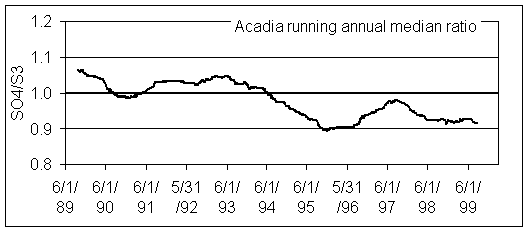Figure 2.  Running annual median ratio of SO4/S3 at Acadia National Park.  Data collected before 3/18/89 are excluded.

Select Western Sites

Rather than focusing on individual sites, we can follow the same procedure for the entire network.  However, care must be taken because of two problems in the IMPROVE data set.

1.   For samples collected between 1992-1994, the sulfur measured on Teflon was sometimes significantly less than the sulfate measured on nylon.  This only occurred at Eastern sites, and predominantly in June, July, and August.  The phenomenon has never been fully explained, but is probably produced by a combination of very high relative humidity, sulfur present predominantly as hygroscopic sulfuric acid, and high face velocities.  With these conditions, some of the sulfate is thought to migrate out of the analytical region examined by x-ray analysis.  In 1995, the face velocity at Eastern sites was decreased by 35% by increasing the collection area.  After that change, the discrepancy was seen on only one day in 1995 in three nearby sites.  The problem is discussed further in Appendix I.  To sidestep this problem, we will focus on Western sites.  For calculation, it was easier to use a preset grouping of all Western sites minus five sites in California with major marine or major nitrate. This is referred to as “Select Western.”

2.   There was a major analytical problem with sulfate on nylon during the initial five quarters on the network.  For some reason, some of the sulfate values reported by the ions laboratory were anomalously low, often near zero even at Eastern sites.  The problem was not observed for samples collected after 3/18/89.  This is discussed more fully in Appendix II.  The problem concentrations were removed from the database, but because this may indicate analytical problems for all samples during this period, we will not consider comparisons before 3/18/89.

Figure 3 shows the running median ratios for quarterly and annual periods.  The overall trend is a decrease in the ratio over the decade.  However, there appears to be actually two periods, separated at about 6/1/93.  Between 6/1/93 and 2/28/00, there is no trend in either plot, although the average ratio is less than unity (0.97).  No reason for the apparent change at 6/93 has been found.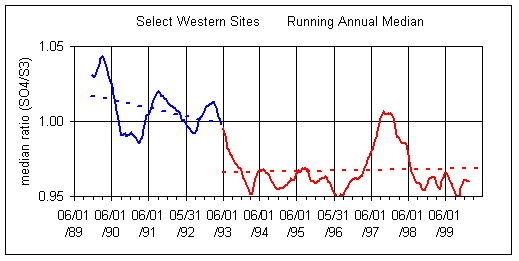Figure 3.  Running quarterly and annual median for Select Western sites (all Western sites except 5 in California).  Both plots split the data into two time periods, before 6/1/93 and after 6/1/93.

Calibration Bias vs. Artifact Bias (Slope vs. Intercept)

The ratio of sulfate/sulfur will depend on both the relative calibration of the two analytical systems (PIXE for Teflon filters and IC for nylon filters) and the artifact subtraction for the two variables.  In order to isolate these two factors, it is better to examine the slope and intercept of the regression between the two variables.  The slope is a measure of the relative bias in the calibration of the two analytical systems.  For a single site, this slope will also include any bias in the flow rate calibration between the A and B modules.  For multiple sites, the flow rate biases will presumably cancel, leaving the relative bias of the two analytical systems.  The intercept primarily reflects the bias in the artifact subtraction.  Thus a trend in artifact subtraction would result in a trend in the intercept but not in the slope.  This argument assumes that the relationship is linear.  The typical r2 of 0.98 indicates that there is a linear relationship.

Figure 4 shows the slope of SO4 vs S3 for the Select Western sites for each quarter since summer 1989.  Overall, the slopes are slightly greater than unity (1.02 ±0.03), indicating the calibration for sulfate gives a slightly higher value than that for sulfur.  The important feature is the trend:  there has been no significant trend in the slope between 1989 and 1999.

Figure 5 shows the intercept for the same data set.  The error bars reflect the uncertainties in the intercept.  For the decade, there is clearly a downward trend in the intercept.  The decrease is about 2.5 ng/m3 per year.  However, for seasons after 9/1/93, the trend is much smaller, dropping to 1 ng/m3 per year.Figure 4.  Slope of SO4 on nylon and S3 on Teflon for each season.  The slope reflects the relative bias in the two analytical systems.  The slope has been relatively constant since summer 1989, with an average value of 1.01.  The trend in the slope has a statistically insignificant decrease of 0.1% per year.  With a typical r2 of 0.98 and about 500 samples per point, the uncertainty for each point is approximately 0.01.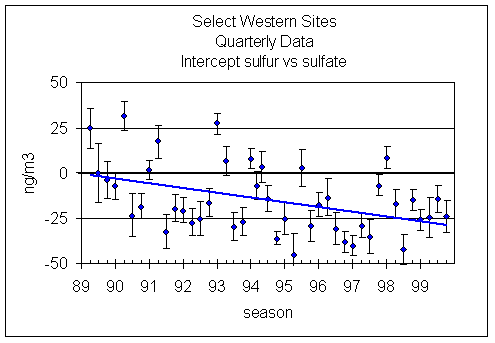Figure 5.  Intercept in regression of SO4 and S3 for each season.  (A negative intercept means the S3 is larger.)  The uncertainty in each intercept is shown.  The downward trend in the intercept is 2.5 ng/m3 per year.

Trends in Artifact Subtraction

The artifact in the data processing is expressed as mass per filter.  This is subtracted by the mass per filter on the sample and the difference is divided by the volume to give concentrations.  For this report, it is clearer to express the artifact as ng/m3 by dividing by the normal volume.

Sulfur on Teflon.  In the CNL system, a clean Teflon filter is used to generate the x-ray background that is removed from the x-ray spectrum.  Thus any sulfur in the filter media will be removed.  The excess sulfur in field blanks is due to any contamination in handling.  Figure 6 shows the distribution of sulfur concentrations on Teflon field blanks.  A zero concentrations means that sulfur was not detected.  None of the measurements were significantly about the minimum detectable limit.  Because the sulfur in field blanks is so near the minimum detectable limit, we have chosen not to subtract any artifact from sulfur.  If one were to subtract an artifact, the best choice would be the median value of 2 ng/m3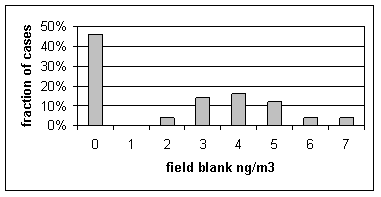Figure 6.  Distribution of sulfur concentrations on Teflon field blanks.  A concentration of zero indicates that the concentration was below the minimum detectable limit.

Sulfate on nylon.  The situation with nylon filters is somewhat different.  Any sulfate in the medium that can be dissolved will be measured as sulfate.  Thus, the sulfate on nylon field blanks is considerably larger, with seasonal median values ranging from 14 to 73 ng/m3.  The global median value over the decade has been 50 ng/m3.  The median standard deviation of the field blanks has been 16 ng/m3.  Because the sulfate artifacts are larger, these are subtracted.

Figure 7 shows the sulfate concentrations of all nylon field blanks since 1989.  The reported masses were divided by the standard volume to give equivalent ng/m3.  Note that about 9/94, scatter of concentrations significantly decreased.  However, even after that point there were two periods of elevated concentrations.  During the 1997 period of elevated field blank, there was a significant change in the relationship with S3.  If fact, after subtracting this large artifact, the agreement with S3 was better than during the periods with lower field blanks.  As will be discussed, to get the best agreement we would have to assume that the true sulfate artifact was zero during the period with small field blank sulfate but equal to the median field blank during the high period.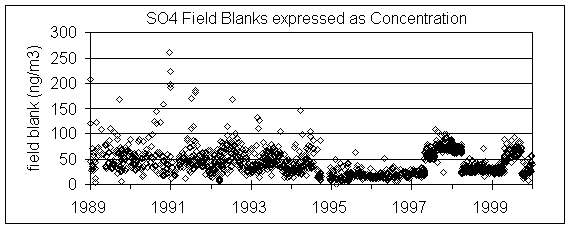Figure 7.  Sulfate on individual nylon field blanks, converted to equivalent concentrations assuming the standard volume.

Figure 8 shows the artifacts for sulfate on nylon used in the data base.  Before 9/94 they were calculated from the mean field blank, with outliers removed.  After this date, they were calculated from the median field blank with no samples removed.  The error bars are the standard deviations of the field blanks.  If medians had been used during the early period, the artifacts would have been reduced by about 10 ng/m3.  Considering the uncertainties during this period, we chose not to recalculate the concentrations using the median as the artifact.Figure 8.  Seasonal artifact in sulfate on nylon.  Before 9/94 they were calculated from the mean field blank, with outliers removed.  After this date, they were calculated from the median field blank with no samples removed.  The error bars are the standard deviations of the field blanks.

Effect of Artifact Bias on the SO4/S3 Ratio

If a and b are the slope and intercept, then the relationship between SO4 and S3 is

SO4 = a * S3 + b.

Assuming the slope differs from unity by δa, the ratio is given by

r = SO4/S3 = a + b/S3 = 1 + ( δa + b/S3 ).

It is possible to separate the slope and intercept components (i.e. calibration and artifact components) by examining different percentiles of the data.  In this section we will calculate the ratios for all data and also for samples with the lower quartile of sulfate.  Thus if the intercept  (artifact) were the major contributor,  the ratio for the lower quartile would differ from unity much more than the ratio for all data.  We will examine the seasonal median ratios for the Select Western sites.

We will consider the ratios in the database using the standard artifact, plus ratios that would occur if we had defined the artifact slightly differently.  This will include subtracting an artifact of 6 ng/m3 for all S3 concentrations, dropping all artifacts, and reducing the sulfate artifacts for samples collected after 1993.

Figure 9 to Figure 12 show the ratio of SO4/S3 for each quarter since summer 1989.  The line with squares is the median ratio for all samples in the quarter.  The thick line, labeled “median ratio-low,” is the median ratio only for those samples that are in the lower quartile of SO4 concentrations.

Figure 9 uses the artifact that was calculated from the field blanks.  The ratios for the lower quartile are consistently lower than those for all samples, especially after summer 1993.  As with the intercept analysis, this suggests that at low concentrations sulfate on nylon is significantly lower than sulfate on Teflon.  That is, if the artifact of sulfate on nylon were to be decreased, or the artifact of sulfur on Teflon were to be increased, the ratios would be closer to unity and the discrepancy between all samples and the lower quartile would be reduced.

Figure 10 subtracts an artifact of 2 µg/m3 from every sulfur concentration.  This is the median of the Teflon field blanks.  The changes between the plots are all small, suggesting that subtracting a small artifact from sulfur does not measurably improve the situation.

Figure 11 shows the artifacts used in the calculation of sulfate concentrations.  The blank SO4 sharply decreased in fall 1994 and significantly increased in 1997.  If the nylon artifact is assumed to be zero, then the ratios are much closer to unity and the quartile effect is small for most of the seasons after 1994 with low blank sulfate.  For all other cases, the ratios are much larger than unity, indicating that there really is an artifact.  Suppose we arbitrarily revise the artifacts calculated from the field blanks.  For most seasons, the artifact was decreased by 5 ng/m3.  This has a very small effect on the ratio.  For most seasons after 6/1/93, the artifact was decreased by 20 ng/m3.  The exception was in the three quarters in 1997 when the blanks had very high sulfate.  The effect of this is much larger.

Figure 12 shows the ratios after reducing the sulfate artifacts to the values shown in Figure 11 and after subtracting 6 ng/m3 from S3.  This is an ad hoc procedure to give better agreement.  This gives the best agreement, but there is no data to justify this procedure.  However, it does show that the trend is very sensitive to slight changes in the artifact.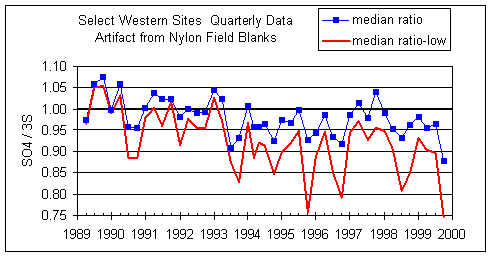Figure 9.  Quarterly ratio of SO4/S3 using the SO4 artifact as calculated from nylon field blanks and zero artifact for S on Teflon.  The upper line is the median ratio for all samples.  The “median ratio-low” line is the median ratio only for those samples that are in the lower quartile of SO4 concentrations.  The lower quartile samples will be much more affected by artifact subtraction for both sulfate and sulfur.Figure 10.  Same as Figure 9, except an artifact of 2 ng/m3 is removed from every S concentration.  The net effect is to shift all ratios up slightly.  However the large discrepancy between all samples and the lower quartile remains.

Figure 11.  SO4 artifacts from nylon field blanks.  The closed diamonds show the artifacts used in the database.  The blank SO4 sharply decreased in fall 1994 and significantly increased in 1997.  The open diamonds show arbitrarily revised artifacts.  For all seasons before 6/1/93, the artifact was not changed.  For most seasons after 6/1/93, the artifact was decreased by 20 ng/m3.  The exception was in the three quarters in 1997 when the blanks had very high sulfate.Figure 12.  Same  as Figure 9, except the nylon SO4 artifact is revised as shown in Figure 11 and an artifact of 6 ng/m3 is subtracted from S3.  This gives much better agreement between SO4 and S3.

There are two methods of evaluating the artifact subtraction.  The first is to examine the lower end of the sulfur-sulfate regression plot.  This method compares the relative artifact subtraction.  With this method is that it is not possible to determine which variable has an artifact bias.  The second method is to examine the lower end of distributions for each variable.  The assumption here is that the concentrations should approach a small value.  Assuming that there is a small baseline background, this will not be quite zero.  It is possible to examine trends here, but the results are open to debate.  Namely, if the trend is up or down, does this indicate an analytical trend or an ambient trend?

Figure 13 and Figure 14 show the paired concentrations for samples where the sulfate on either filter is less than 300 ng/m3.  Data from all network sites are included.  All years are from March to the following February.  In 1991 and 1997, the two measurements lie symmetrically about the 1:1 line.  In 1989, the sulfate (nylon) is slightly higher.  In all other years, the sulfate on nylon is about 20 ng/m3 below S3.  This is about 3 times the concentration of S3 on Teflon blank filters.  If this had been subtracted, the differences would be about 15 ng/m3.  It is difficult to discern any trend.

Figure 15 and Figure 16 show the distribution of S3 and SO4 by year for the lowest 2% of samples.  The trends in minimum for the year and for three percentile concentrations are shown in Figure 17.  In all cases, the difference changes by about 30 ng/m3 between 1989 and 1999.  The biggest difference is in the minimum concentration for the year.  For S3, there is no trend, with minimum concentrations averaging 30 ng/m3.  For SO4, the average minimum is also around 30 ng/m3, but there is a significant trend going from 50 ng/m3 in 1989 to 10 ng/m3 in 1997, and rising slightly in the past two years.  For the various percentiles, both variables show decreasing trends, but the decrease for SO4 is always larger than for S3.

Note that all the very low concentrations in 1997 occurred around 1/10/98, at several sites from Northern California to Washington.  January 7-14, 1998 was the cleanest week in the 12-year period of record.

On the basis of distributions, it is clear that the sulfur on Teflon in the database is slightly larger than sulfate on nylon for very low concentrations.  However, it is very difficult to determine which variable is better.

Conclusions

There is a disagreement between sulfate on nylon and sulfur on Teflon for low concentrations.  For most years, sulfate on nylon is lower than sulfur on Teflon.  The important trend is that the difference has increased by about 30 ng/m3.  For a network median sulfate concentration of 800 ng/m3, this corresponds to a 4% change in the slope.  This is about what is observed.  The most important question is why is there a trend?  The methodology of subtracting field blanks has been consistent.  The analytical systems and protocols have not changed.  Maybe there is a worldwide increase in non-sulfate sulfur.  That would make a great paper.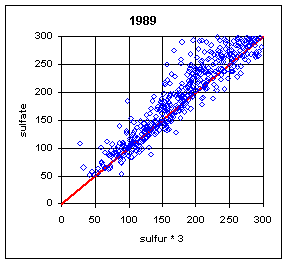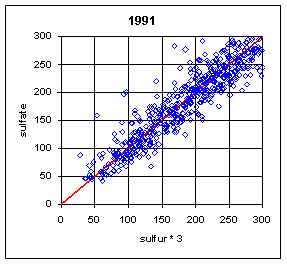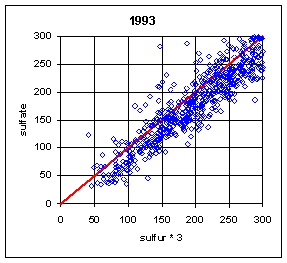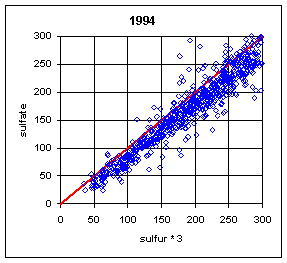Figure 13.  Low-end comparison of SO4 and S3 for years 1989-94.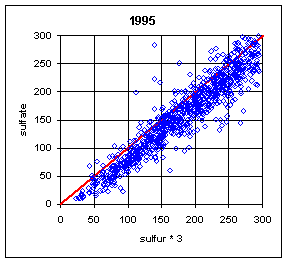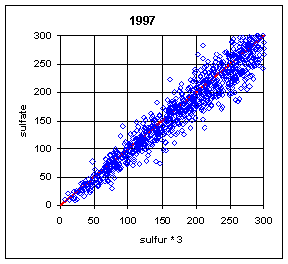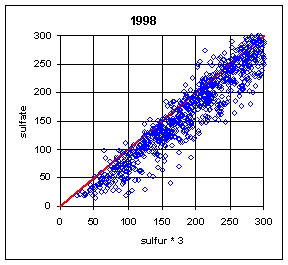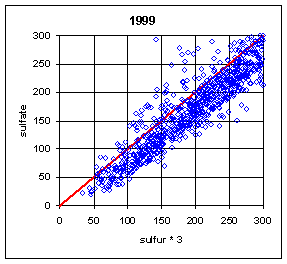Figure 14.  Low-end comparison of SO4 and S3 for years 1995-99.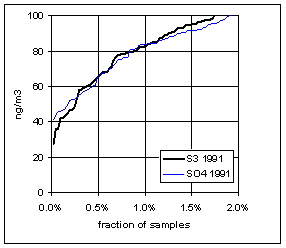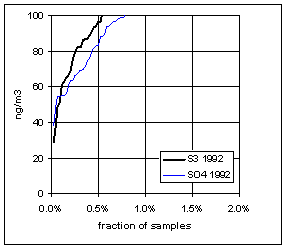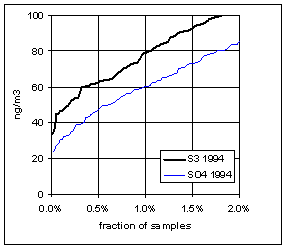Figure 15.  Low-end distribution of S3 and SO4 for 1989-94.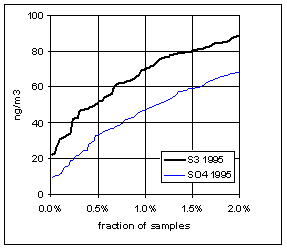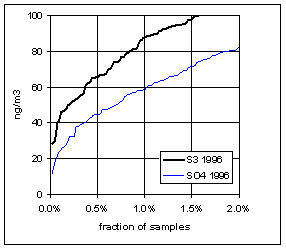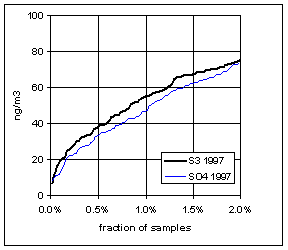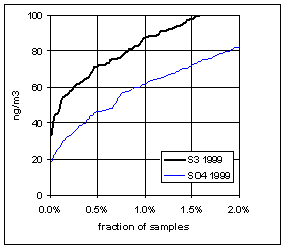Figure 16.  Low-end distribution of S3 and SO4 for 1995-99.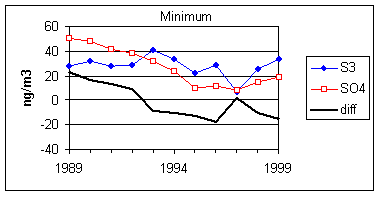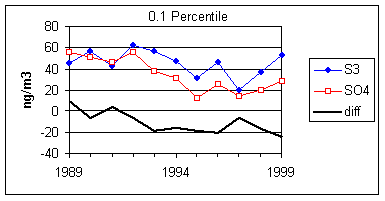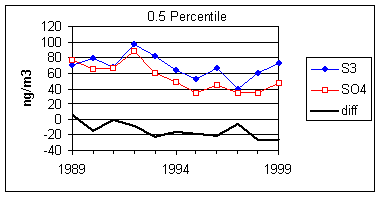Figure 17.  Trends in the low-end distributions of S3 and SO4.  The heavy line is the difference between SO4 and S3.

Appendix I:  Loss of Sulfur on Teflon Filters

Normally, the sulfur on Teflon and the sulfate on nylon have correlated extremely well.  One exception was during the summers (June-August) of 1992-94.  During this period, a large number of samples at Eastern sites had much lower sulfur on Teflon compared to sulfate on nylon.  The phenomenon is shown in Figure 18.  The phenomenon was never observed in the West, and rarely in other months in the East.  The mechanism has never been fully understood, but probably is associated with very high relative humidity, acidic sulfate particles, and high face velocities.  Under certain conditions, the sulfuric acid becomes saturated and migrates outside the x-ray analysis area.  When the collection area was changed from 2.2 cm2 to 3.5 cm2 (the full 25 mm filter) in 1995, thereby reducing the face velocity from 170 cm/s to 110 cm/s, the discrepancy disappeared, except for one day in August 1995.

There were no differences between XRF and PIXE on the Teflon filters.  Both XRF and PIXE look at the central region of the filter, with PIXE looking at a slightly smaller area.  The conclusion is that the sulfate must have migrated even beyond the XRF area.

The periods with largest differences between S3 and SO4 also had the largest differences between organic by hydrogen and organic by carbon.  The calculation of organic by hydrogen will significantly underestimate organic mass if sulfur is primarily present as sulfuric acid.  If the sulfuric acid had migrated out of the analysis area, neither the sulfur nor the hydrogen would be counted.  Only the remaining particles would affect the calculation.  The inference is that even the remaining particles were acidic.

Examination of the relative humidity at Shenandoah during the summer of 1993 showed that the samples with differences over 1 µg/m3 occurred during periods of higher RH:  an average of 5 of the 24 hours had RH at 100%, compared to 2 hours for samples with smaller differences.

In a special study at Great Smoky Mountains in summer 1994, 4h and 12h measurements were made with Teflon and nylon.  All modules had a denuder except the 12h Teflon.  The 4h Teflon agreed with the 4h nylon and 12h nylon, but the 12h Teflon sometimes gave lower concentrations.  Since the face velocity, RH, and acidity were the same for both 12h and 4h Teflon filters, some other factor had to be involved.  There are two possible hypotheses.  One is that the migration takes several hours.  A more likely hypothesis is that the carbonate denuder moderated the RH at the filter.  The amount of water that the denuder could retain in finite, but it might reduce RH when the ambient RH is at 100% and later add some water when the ambient RH decreases.  The conclusion would then be that the RH must be at almost 100%.

A group of Teflon filters with and without large discrepancies were analyzed by IC after the XRF and PIXE analyses.  The results are shown in Figure 19.  Note that Teflon filters are routinely reanalyzed by PIXE and XRF and show that no sulfur is lost during the PIXE analysis.  This is borne out by the IC analysis on the Teflon filters with no discrepancy;  the IC on Teflon agrees with PIXE and Teflon and IC on nylon.  There were some cases, especially those with very high sulfate, where the IC on Teflon agreed with the IC on nylon.  In these cases, the sulfate must have remained on the filter but simply migrated outside the view of PIXE.  However, there are many cases when the IC values on Teflon were intermediate between the PIXE on Teflon and the IC on nylon.  In these case, some of the sulfate remained on the filter but migrated further out, and some of the sulfate disappeared from the Teflon filter completely.

This mixture of effects was also obtained by scanning the entire filter using a focused PIXE beam.  In this case, sometimes sulfur was observed on the outer portions of the filter, but on other filters no additional sulfur was observed.

It should also be noted that this effect could appear again.  It was observed at Washington DC during the 1992-94 period despite having the larger 3.5 cm2 collection area.   It was also observed on one day in 1995 despite the larger collection area.

The phenomenon is not limited to the IMPROVE sampler.  Differences were observed in CASTNet samplers in the East using Teflon filters with very high face velocities.   The Teflon filters at that time were analyzed by UCD.Figure 18.  Comparison of sulfur and sulfate.  The plots on the left are for all remote Eastern sites in summer (June-August).  (This excludes Washington DC).  There are a few cases with sulfur lower than sulfate before 1992.  During the period from 1992 to 1994, a significant number of samples had much lower sulfur on Teflon than sulfate on nylon.  After 1994, there was only one day with lower sulfur, at three sites in Virginia and West Virginia.  The upper right side is for Eastern sites outside of summer.  The only samples with differences were in the first week of September or the last week in May.  The lower right plot is for Western sites in summer; there were no cases with significant differences.Figure 19.  Comparison of IC sulfate on nylon with IC sulfate and PIXE sulfur on Teflon.  The IC analysis was performed on the Teflon filters after the XRF and PIXE analyses.

Appendix II:  Analytical Errors on Sulfate by Ion Chromatography

For some reason, the sulfate concentration was occasionally underreported by RTI during the initial five quarters.  Figure 20 shows the fraction of samples invalidated for each sampling period during the five quarters.  Figure 21 shows that the problem was not associated with the concentration of sulfate; the distribution of sulfur on Teflon was the same for valid and invalid sulfate.  Although the sulfate values in the database after validation may be acceptable, all comparisons before 3/18/89 are suspect.Figure 20.  Fraction of  samples with invalid (low) sulfate on the nylon filter and apparently normal nitrate.Figure 21.  Distributions of sulfur for samples collected between March 1988 and February 1989.  One distribution is for samples with valid sulfate measurements and the other for invalid sulfate.